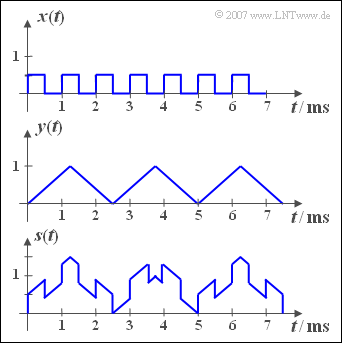# Exercise 2.1Z: Sum SignalRectangular signal, triangular signal, sum signal

The adjacent diagram shows the periodic signals  ${x(t)}$  and  ${y(t)}$,  from which the sum   ${s(t)}$  – sketched in the lower diagram – and the difference  ${d(t)}$  are formed.

Furthermore, in this task we consider the signal  ${w(t)}$, which results from the sum of two periodic signals  ${u(t)}$  and  $v(t)$ .  The base frequencies of these signals are

• $f_u = 998 \,\text{Hz},$
• $f_v = 1002 \,\text{Hz}.$

That is all we know about the signals  ${u(t)}$  and  $v(t)$.

Hints:

### Questions

1

What is the period duration  $T_x$  and the basic frequency  $f_x$  of the signal  ${x(t)}$?

 $f_x\ = \$   $\text{kHz}$

2

What is the period duration  $T_y$  and the basic frequency  $f_y$  of the signal  ${y(t)}$?

 $f_y\ = \$   $\text{kHz}$

3

Determine the basic frequency  $f_s$  and the period duration  $T_s$  of the sum signal  ${s(t)}$.
Verify your results with the help of the sketched signal.

 $T_s\ = \$   $\text{ms}$

4

What is the period duration  $T_d$  of the difference signal  ${d(t)}$ ?

 $T_d\ = \$   $\text{ms}$

5

What is the period duration  $T_w$  of the signal  ${w(t)} = {u(t)} + v(t)$?

 $T_w\ = \$   $\text{ms}$

### Solution

#### Solution

(1)  The following applies to the rectangular signal:  $T_x = 1 \,\text{ms}$   ⇒

$$f_x \hspace{0.15cm}\underline{= 1 \, \text{kHz}}.$$

(2)  The following applies to the triangular signal:  $T_y = 2.5 \,\text{ms}$  und

$$f_y \hspace{0.15cm}\underline{= 0.4\, \text{kHz}}.$$

(3)  The basic frequency  $f_s$  of the sum signal  $s(t)$  is the greatest common divisor  $f_x = 1 \,\text{kHz}$  and  $f_y = 0.4 \,\text{kHz}$.

• From this follows  $f_s = 200 \,\text{Hz}$  and the period duration  $T_s\hspace{0.15cm}\underline{ = 5 \,\text{ms}}$.
• This is also evident from the graphic on the information page.

(4)  The period duration  $T_d$  does not change compared to the period duration  $T_s$,  if the signal  ${y(t)}$  is not added but subtracted:

$$T_d = T_s \hspace{0.15cm}\underline{= 5\, \text{ms}}.$$

(5)  The greatest common divisor of  $f_u = 998 \,\text{Hz}$  and  $f_{v} = 1002 \,\text{Hz}$  is  $f_w = 2 \,\text{Hz}$.  The inverse of this gives the period duration  $T_w \hspace{0.15cm}\underline{= 500 \,\text{ms}}$.